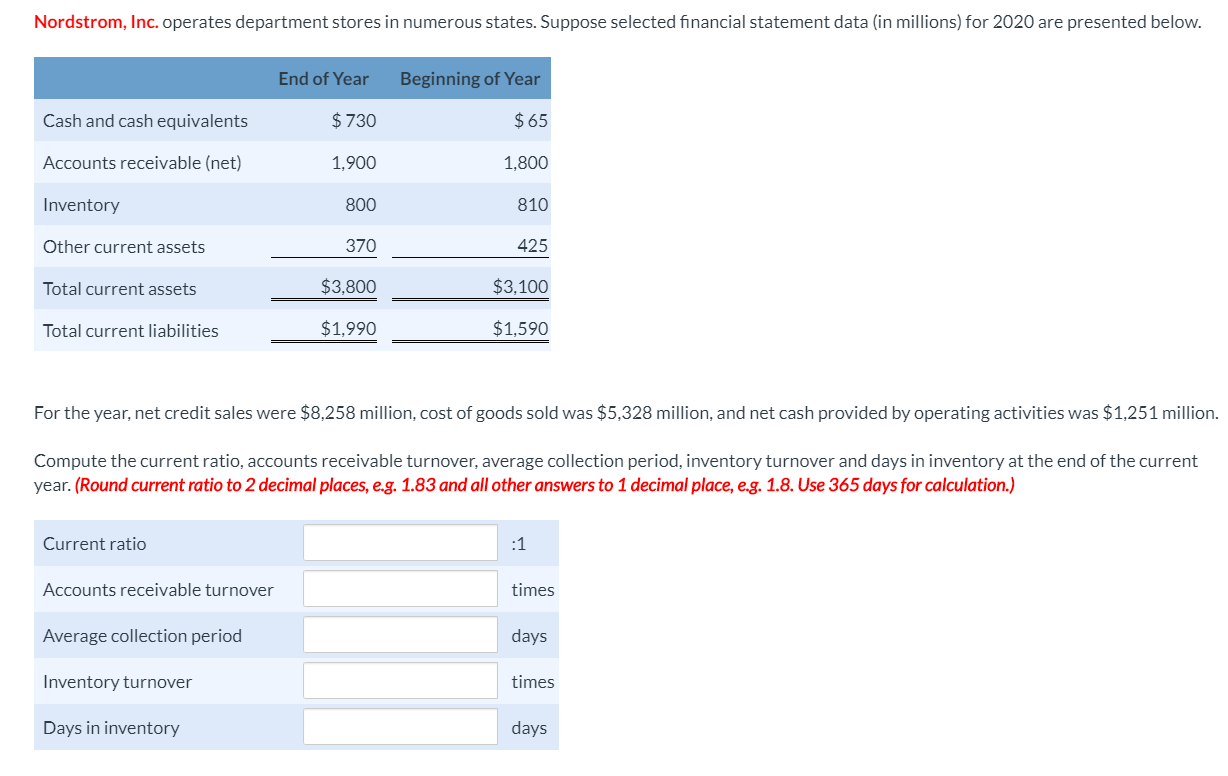# Nordstrom, Inc. operates department stores in numerous states. Suppose selected financial statement data (in millions) for 2020 are presented below. End of Year Beginning of Year $730$65 Cash and cash equivalents Accounts receivable (net) 1,900 1,800 Inventory 800 810 370 425 Other current assets $3,800$3,100 Total current assets $1,990$1,590 Total current liabilities For the year, net credit sales were $8,258 million, cost of goods sold was$5,328 million, and net cash provided by operating activities was $1,251 million. Compute the current ratio, accounts receivable turnover, average collection period, inventory turnover and days in inventory at the end of the current year. (Round current ratio to 2 decimal places, e.g. 1.83 and all other answers to 1 decimal place, e.g. 1.8. Use 365 days for calculation.) Current ratio :1 Accounts receivable turnover times Average collection period days Inventory turnover times Days in inventory days Questionhelp_outlineImage TranscriptioncloseNordstrom, Inc. operates department stores in numerous states. Suppose selected financial statement data (in millions) for 2020 are presented below. End of Year Beginning of Year$730 $65 Cash and cash equivalents Accounts receivable (net) 1,900 1,800 Inventory 800 810 370 425 Other current assets$3,800 $3,100 Total current assets$1,990 $1,590 Total current liabilities For the year, net credit sales were$8,258 million, cost of goods sold was $5,328 million, and net cash provided by operating activities was$1,251 million. Compute the current ratio, accounts receivable turnover, average collection period, inventory turnover and days in inventory at the end of the current year. (Round current ratio to 2 decimal places, e.g. 1.83 and all other answers to 1 decimal place, e.g. 1.8. Use 365 days for calculation.) Current ratio :1 Accounts receivable turnover times Average collection period days Inventory turnover times Days in inventory days fullscreen

### Want to see this answer and more?

Experts are waiting 24/7 to provide step-by-step solutions in as fast as 30 minutes!*